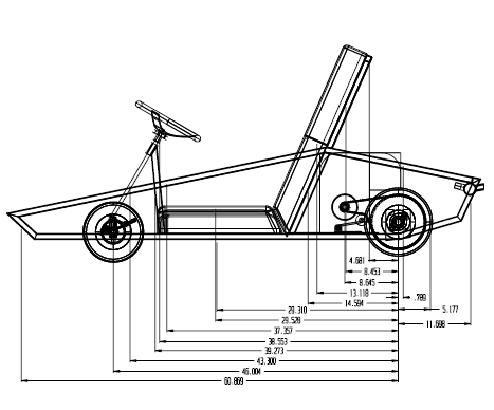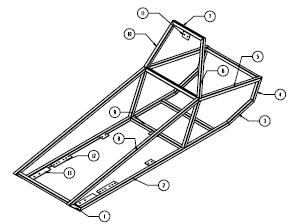# Go Kart Weight Distribution Calculations

The weight distribution calculator sums up all the weight data and calculates the center of gravity (or in this case how much percentage of weight is over the tires).

The center of gravity is calculated by summing the moments about the rear axel. So all the radial moment arms and wieghts need to be either calculated or measured.The diagram above has the tubes numbered.  Example Tube7 is the top tube in the roll bar and tube2 is the long horzontal tube on the frame side.

To change the position of the center of gravity, start changing the positions of the larger parts like the engine, and the person.

(Note:  All items behind the rear wheel have a negative sign, or negative radial value)

The actual theory of Center of Gravity is discussed and derived in the Go Kart Building 301.  What you will find out is that placing the components correctly is critical to go kart performance and handling.